Date: Sat, 5 Sep 1998 09:14:11 -0600 (CST)
Subject: triminoes

Name: Roxanne

Level: Secondary

Question:
I am doing an investigation about a game called triminoes (like dominoes). The game is played using triangular pieces of card. Each card has 3 numbers on it. I have to investigate the relationship between the number of trimino cards in a set and the largest number on the cards. I found;
 largest no. used 0 1 2 3 4 no. of trimino cards 1 4 10 20 35
I was ginen the formula for this which is: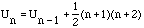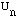= no. of trimino cards n = largest no.

I don't know how to get to this equation I think it has something to do with triangle numbers!

Hi Roxanne,

The use of triangular numbers in this problem is possible but there is a more direct way to come up with an expression for the number of trimino cards.
Suppose that k is the number of numbers that you have, so k=n+1, and you want to count the number of cards that you can make.

• There are cards with three different numbers on them. The number of such cards is the number of ways of choosing three numbers from k numbers, that is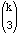.
• There are cards with the same number three times. The number of such cards is the number of ways of choosing one numbers from k numbers, that is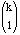• There are cards with one number twice and another number once. There are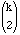ways of choosing two numbers from k and once you have the two numbers there are two triminos you can make, one with two copies of the first number and one of the second and another with one copy of the first number and one of the first.
Hence there are 2cards with exactly two distinct numbers on them.
Thus, altogether there are++2triminos you can make with k numbers.

You can use Pascals identity that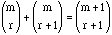to simplify the expression above.++2=+++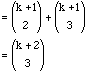Since k=n+1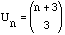Note: This is clearly the expression that you are looking for since it agrees with,

but I find it a little unusual. If triminoes are like dominoes and the numbers or markings are only on one side then I would think that the two triminoes shown here are different.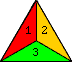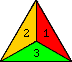and yet in the development above they are counted as the same trimino.

Cheers,
Penny

Go to Math Central